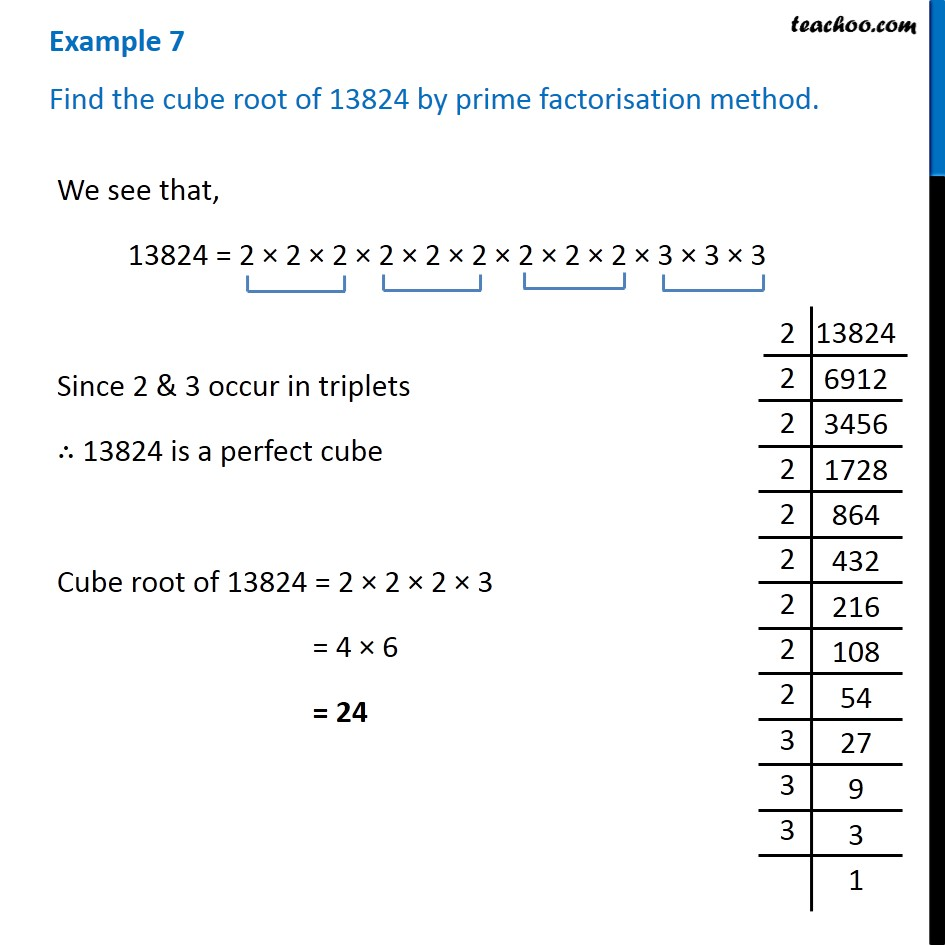1. Chapter 7 Class 8 Cubes and Cube Roots
2. Concept wise
3. Finding cube root by prime factorisation method

Transcript

Example 7 Find the cube root of 13824 by prime factorisation method. We see that, 13824 = 2 × 2 × 2 × 2 × 2 × 2 × 2 × 2 × 2 × 3 × 3 × 3 Since 2 & 3 occur in triplets ∴ 13824 is a perfect cube Cube root of 13824 = 2 × 2 × 2 × 3 = 4 × 6 = 24

Finding cube root by prime factorisation method